# How to find the percentile if with criteria in Excel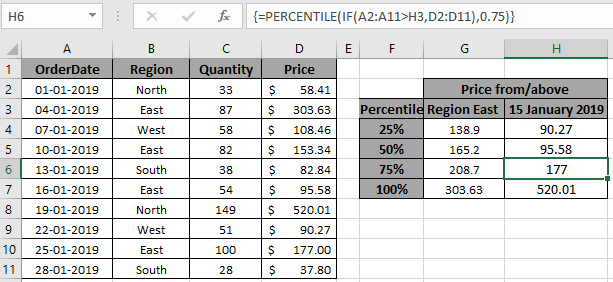In this article, we will learn How to How to find the percentile value if with given criteria in Excel

Scenario:

In simple words, when working with a long scattered dataset, sometimes we need to find the percentile of numbers with some criteria over it. For example, finding the percentile of salaries in a particular department or having multiple criterias over date, names, department or can even numbers data like salaries below value or quantity above value. For this you have to manually extract required numbers and then calculate the percentile of an array with criteria. Use of IF function with array formulas.

How to solve the problem?

You must be thinking how is this possible, to perform logical operations over table arrays using IF function. IF function in excel is very useful, It will get you through some difficult tasks in Excel or any other coding languages. IF function tests conditions on array corresponding to required values and returns the result as array corresponding to True conditions as 1 and False as 0

For this problem, we will be using the following functions :

We will be requiring these above functions and some basic sense of data operation. logical conditions on arrays can be applied using logical operators. These logic operators work on text and numbers both. Below here is the generic formula. {  } curly braces is the magic tool to perform array formulas with IF function.

Generic formula:

 { = PERCENTILE ( IF ( (range op crit), percentile_array ), k ) }

Note:  For curly braces ( { } ) Use Ctrl + Shift + Enter when working with arrays or ranges in Excel. This will generate Curly Braces on the formula by default. DO NOT try to hard code curly braces characters.

range : array, where condition applies

op : operator, operation applied

crit : criteria applied on range

percentile_array : array, where percentile be needed

k : kth percentile (for example 33% -> k = 0.33 value as number)

Example :

All of these might be confusing to understand. So, let's test this formula via running it on the example shown below. Here we have data of received products from different regions along with corresponding date, quantity and price. Here we have the data and we need to find the 25th percentile or value corresponding to 25% considering orders which are only received from "East" region. Now you are ready to get the desired result using the below formula.

Use the formula :

 { = PERCENTILE ( IF ( B2:B11 = "East" , D2:D11 ) , 0.25 ) }

Explanation :

1. Region B2:B11 ="East" : checks the values in array region to match with "East".
2. Logical operator returns True for the matched value and False for the unmatched value.
3. Now IF function only returns the price values corresponding to the 1s and False as it is. Below ais the range returned by IF function and received by PERCENTILE funtion.

{ FALSE ; 303.63 ; FALSE ; 153.34 ; FALSE ; 95.58 ; FALSE ; FALSE ; 177 ; FALSE }

1. PERCENTILE function returns the 25% (k=0.25) percentile price for the returned array.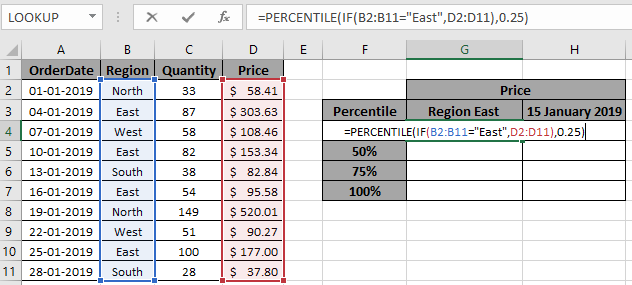Here the arrays are given using the cell reference. Now the price corresponding to the 25% of the array is given below.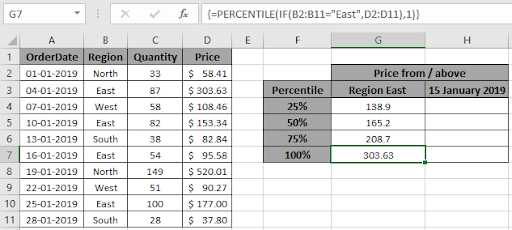As you can see, price comes to be 138.9 , from the three orders from "East" having price values 303.63, 153.34 and 95.58. Now get the price value having different percentile just by changing the kth value to 0.5 for 50%, 0.75 for 75% and 1 for 100% percentile value.As you can see, we have all the percentile values corresponding to given criteria. Now use the formula with Date value as criteria.

percentile if with date criteria in Excel :

Date as criteria is a tricky thing in Excel. As Excel stores date values as number. So if date value is not recognized by excel then formula return error. Here we need to find the 25% percentile value having orders received after 15 January 2019.

Use the formula:

 { = PERCENTILE ( IF ( A2:A11 > H3 , D2:D11 ) , 0.25 ) }

> : greater than operator applied over the date array.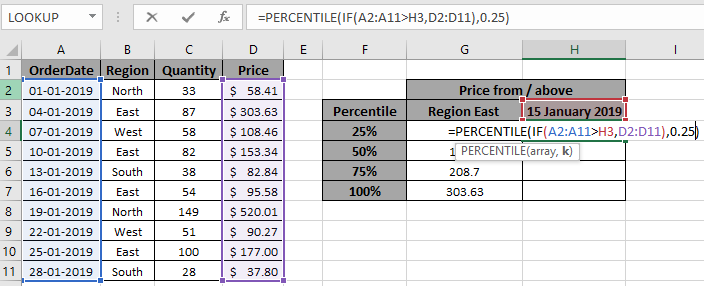Here the arrays are given using the cell reference. Now the price corresponding to the 25% of the array is given below.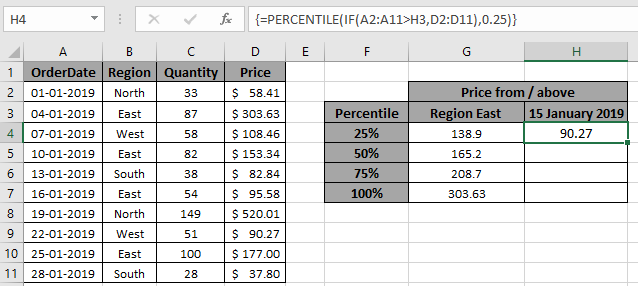As you can see, 90.27 is the 25th percentile value having date after 15 Jan 2019. Now get the percentile for the different kth value.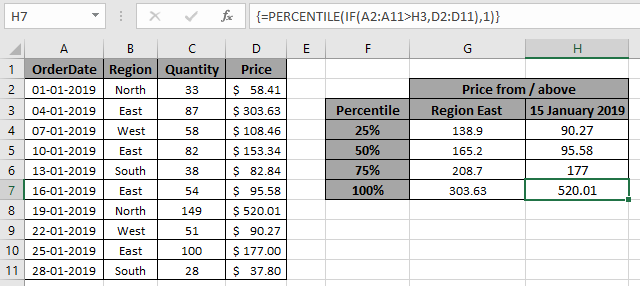Here are all the percentile values as results

Here are all the observational notes regarding using the formula.

Notes:

1. The percentile_array in the formula only works with numbers.
2. DO NOT hard code curly braces with formula.
3. If the formula returns #VALUE error, check for the curly braces must be present in the formula as shown in the examples in the article.
4. Operations like equals to ( = ), less than equal to ( <= ), greater than ( > ) or not equals to ( <> ) can be performed within a formula applied, with numbers only.

Hope this article about How to How to find the percentile if with criteria in Excel is explanatory. Find more articles on Summing formulas here. If you liked our blogs, share it with your fristarts on Facebook. And also you can follow us on Twitter and Facebook. We would love to hear from you, do let us know how we can improve, complement or innovate our work and make it better for you. Write us at info@exceltip.com

Related Articles :

How to use the SUMPRODUCT function in Excel: Returns the SUM after multiplication of values in multiple arrays in excel.

SUM if date is between : Returns the SUM of values between given dates or period in excel.

Sum if date is greater than given date: Returns the SUM of values after the given date or period in excel.

2 Ways to Sum by Month in Excel: Returns the SUM of values within a given specific month in excel.

How to Sum Multiple Columns with Condition: Returns the SUM of values across multiple columns having condition in excel.

Popular Articles :

50 Excel Shortcut to Increase Your Productivity : Get faster at your task. These 50 shortcuts will make you work even faster on Excel.

How to use the VLOOKUP Function in Excel : This is one of the most used and popular functions of excel that is used to lookup value from different ranges and sheets.

How to use the COUNTIF function in Excel : Count values with conditions using this amazing function. You don't need to filter your data to count specific values. Countif function is essential to prepare your dashboard.

How to Use SUMIF Function in Excel : This is another dashboard essential function. This helps you sum up values on specific conditions.

Terms and Conditions of use

The applications/code on this site are distributed as is and without warranties or liability. In no event shall the owner of the copyrights, or the authors of the applications/code be liable for any loss of profit, any problems or any damage resulting from the use or evaluation of the applications/code.